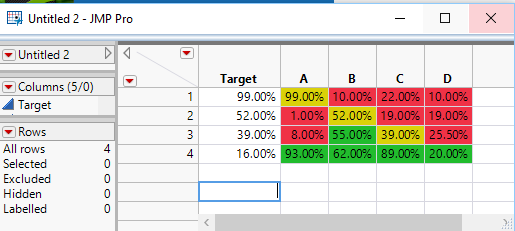Our World Statistics Day conversations have been a great reminder of how much statistics can inform our lives. Do you have an example of how statistics has made a difference in your life? Share your story with the Community!
Choose Language Hide Translation Bar
Highlighted

## Color cells based on multiple conditions

I have written the following code to color the cells based on condition.I need to add one more condition -

if weight is within -2% of Target then need to color cells yellow.How do I do this?

``````dt << New Column("color cells",
Formula(If(:Target> :weight, :weight << color cells("Red", Row()); .,
:weight << color cells("Green", Row()); .)),
Hide(1)
);``````

Thanks

1 ACCEPTED SOLUTION

Accepted Solutions
Highlighted

## Re: Color cells based on multiple conditions

If(
:Target > :weight,
:weight << color cells( "Red", Row() );
.;,
:weight < :Target + (.02 * Abs( :Target )) & :weight > :Target - (.02 *
Abs( :Target )),
:weight << color cells( "Yellow", Row() );
.;,
:weight << color cells( "Green", Row() );
.;
);

Jim
3 REPLIES 3
Highlighted

## Re: Color cells based on multiple conditions

If(
:Target > :weight,
:weight << color cells( "Red", Row() );
.;,
:weight < :Target + (.02 * Abs( :Target )) & :weight > :Target - (.02 *
Abs( :Target )),
:weight << color cells( "Yellow", Row() );
.;,
:weight << color cells( "Green", Row() );
.;
);

Jim
Highlighted

## Re: Color cells based on multiple conditions

Need to script a variation of this logic. I have data in 4 columns and need to compare the value in each cell with the Target value. Also I wouldnt know the column names before hand. How to do this? In simple words,need to compare values in column A,B,C and D with corresponding value in Target column and color code(Green,Yellow or Red) accordingly.

 Target A B C D 99% 1% 8% 93% 10% 52% 55% 62% 22% 19% 39% 89% 18% 33% 25.50% 16% 11% 92% 10% 2%
Highlighted

## Re: Color cells based on multiple conditions

1. If you are going to be working in JSL, you need to take the time to read the Scripting Guide:    Help==>Books==>Scripting Guide

2. Below is a simple script that generates the coloring as you described``````Names Default To Here( 1 );
dt = Current Data Table();

colNamesList = dt << get column names( string );

For( theRow = 1, theRow <= N Rows( dt ), theRow++,
For( theCol = 2, theCol <= N Items( colNamesList ), theCol++,
If(
:Target[theRow] > Column( dt, colNamesList[theCol] )[theRow],
Column( dt, colNamesList[theCol] ) << color cells( "Red", theRow );
.;,
Column( dt, colNamesList[theCol] )[theRow] < :Target[theRow] + (.02 *
Abs( :Target[theRow] )) & Column( dt, colNamesList[theCol] )[theRow] >
:Target[theRow] - (.02 * Abs( :Target[theRow] )),
Column( dt, colNamesList[theCol] ) << color cells( "Yellow", theRow );
.;,
Column( dt, colNamesList[theCol] ) << color cells( "Green", theRow );
.;
)
)
);``````
Jim
Article Labels

There are no labels assigned to this post.